# Python 基础学习一

Python 基础运算符学习。

## 一、算术运算符

SYMBOL OPERATION
+ addition
- subtraction
* multiplication
/ division
% modulo

### python 代码格式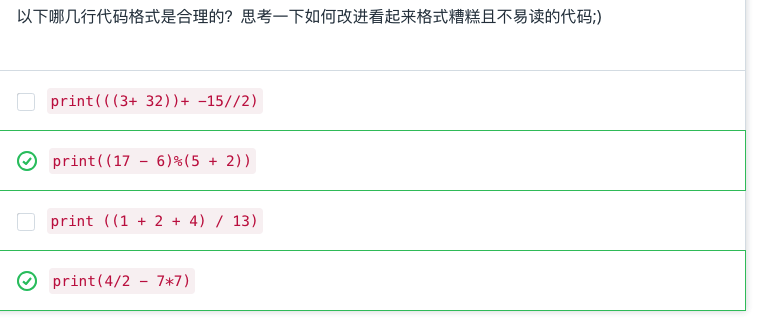print((3 + 32) - 15//2)

print((1 + 2 + 4)/13)

### python 变量命名

1. 只能在变量名称中使用常规字母、数字和下划线。不能包含空格，并且需要以字母或下划线开头。
1. 不能使用保留字或内置标识符，它们在 Python 中具有重要含义，你将在整个这门课程中学到这些知识。python 保留字列表请参阅此处。创建对值清晰描述的名称可以帮助你避免使用这些保留字。下面是这些保留字的简要表格。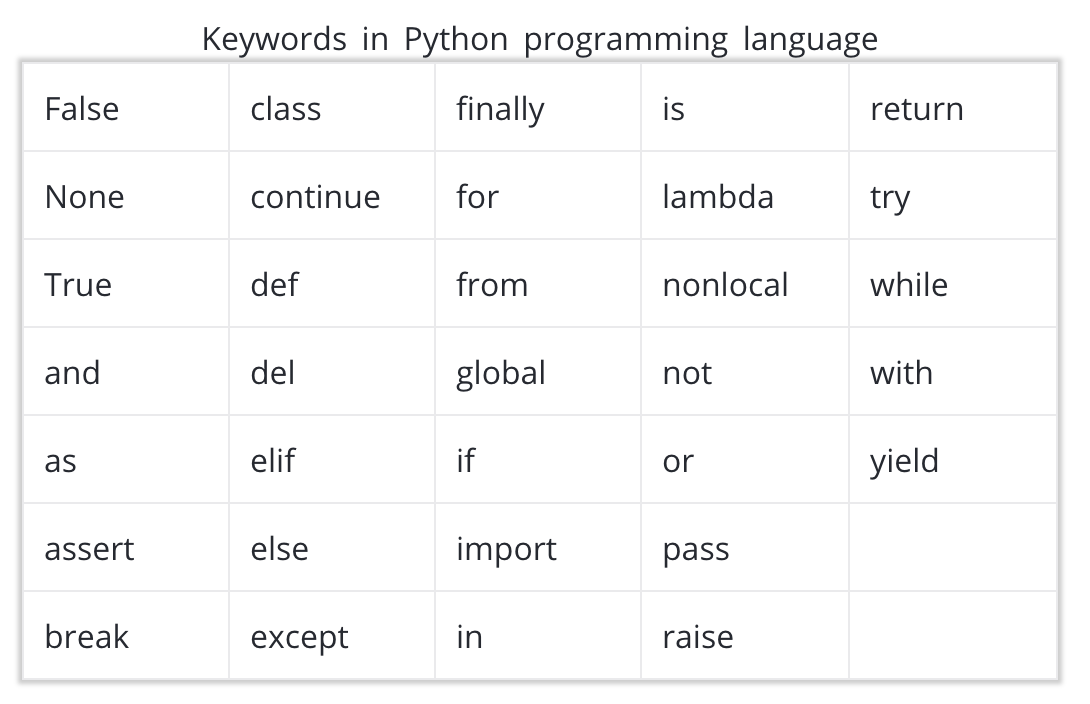1. 在 python 中，变量名称的命名方式是全部使用小写字母，并用下划线区分单词。

my_height = 58
my_lat = 40
my_long = 105

my height = 58
MYLONG = 40
MyLat = 105

### 赋值运算符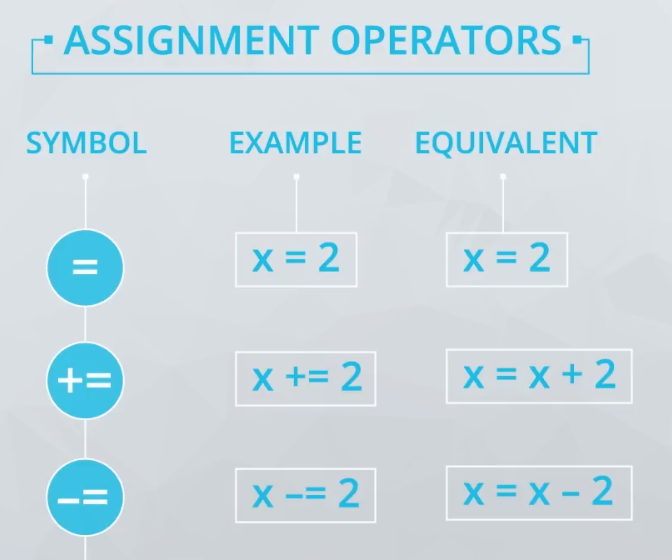### 整数和浮点数

• int - 表示整数值
• float - 表示小数或浮点数值
你可以通过以下语法创建具有某个数据类型的值：
x = int(4.7)   # x is now an integer 4
y = float(4)   # y is now a float of 4.0

你可以使用函数 type 检查数据类型：

>>> print(type(x))
int
>>> print(type(y))
float

因为 0.1 的浮点数（或近似值）实际上比 0.1 稍微大些，当我们将好几个这样的值相加时，可以看出在数学上正确的答案与 Python 生成的答案之间有区别。

>>> print(.1 + .1 + .1 == .3)
False

## 字符串

>>> my_string = 'this is a string!'
>>> my_string2 = "this is also a string!!!"

>>> this_string = 'Simon\'s skateboard is in the garage.'
>>> print(this_string)

### len()函数用法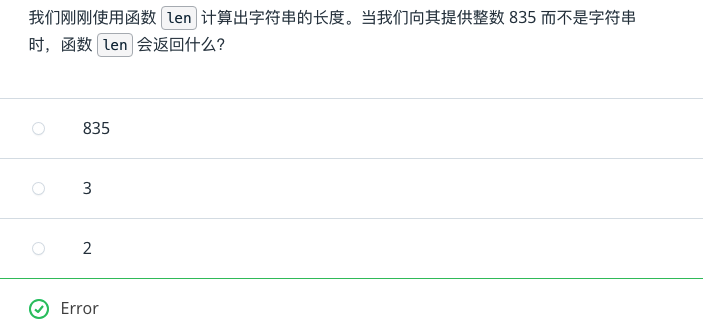## 类型和类型转换

• 1.整型
• 2.浮点型
• 3.布尔型
• 4.字符串
你在之前的视频中，简单了解了 type()，它可以用来检查你所处理的任何变量的数据类型。
>>> print(type(4))
int
>>> print(type(3.7))
float
>>> print(type('this'))
str
>>> print(type(True))
bool

mon_sales = "121"
tues_sales = "105"
wed_sales = "110"
thurs_sales = "98"
fri_sales = "95"

#TODO: Print a string with this format: This week's total sales: xxx
# You will probably need to write some lines of code before the print statement.

sum = int(mon_sales) + int(tues_sales) + int(wed_sales) + int(thurs_sales) + int(fri_sales)

print("This week's total sales:" + str(sum))

## 字符串方法

title()
islower()
count()

print("One fish, two fish, red fish".count('fish'))

len("this")
type(12)
print("Hello world")

typeprint 函数可以接收字符串、浮点型、整型和很多其他数据类型的参数，函数 len 也可以接受多种不同数据类型的参数，稍后你将在这节课中详细了解。

python 中的方法和函数相似，但是它针对的是你已经创建的变量。方法特定于存储在特定变量中的数据类型。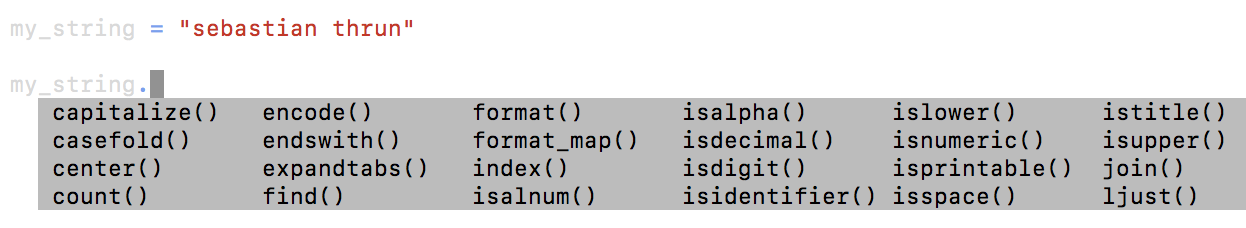>>> my_string.islower()
True
>>> my_string.count('a')
2
>>> my_string.find('a')
3